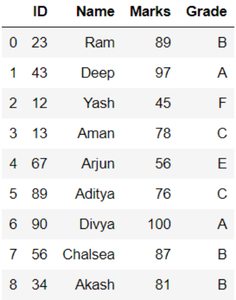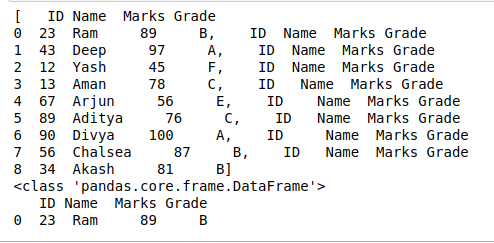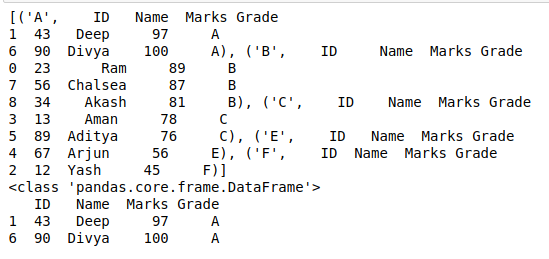Related Articles
Split large Pandas Dataframe into list of smaller Dataframes
• Last Updated : 05 Sep, 2020

In this article, we will learn about the splitting of large dataframe into list of smaller dataframes. This can be done mainly in two different ways :

1. By splitting each row
2. Using the concept of groupby

Here we use a small dataframe to understand the concept easily and this can also be implemented in an easy way. The Dataframe consists of student id, name, marks, and grades. Let’s create the dataframe.

## Python3

 `# importing packages``import` `pandas as pd`` ` `# dictionary of data``dct ``=` `{``'ID'``: {``0``: ``23``, ``1``: ``43``, ``2``: ``12``,``              ``3``: ``13``, ``4``: ``67``, ``5``: ``89``,``              ``6``: ``90``, ``7``: ``56``, ``8``: ``34``},``        ` `       ``'Name'``: {``0``: ``'Ram'``, ``1``: ``'Deep'``,``                ``2``: ``'Yash'``, ``3``: ``'Aman'``,``                ``4``: ``'Arjun'``, ``5``: ``'Aditya'``,``                ``6``: ``'Divya'``, ``7``: ``'Chalsea'``,``                ``8``: ``'Akash'``},``        ` `       ``'Marks'``: {``0``: ``89``, ``1``: ``97``, ``2``: ``45``, ``3``: ``78``,``                 ``4``: ``56``, ``5``: ``76``, ``6``: ``100``, ``7``: ``87``,``                 ``8``: ``81``},``        ` `       ``'Grade'``: {``0``: ``'B'``, ``1``: ``'A'``, ``2``: ``'F'``, ``3``: ``'C'``,``                 ``4``: ``'E'``, ``5``: ``'C'``, ``6``: ``'A'``, ``7``: ``'B'``,``                 ``8``: ``'B'``}``       ``}`` ` `# create dataframe``df ``=` `pd.DataFrame(dct)`` ` `# view dataframe``df`

Output:Below is the implementation of the above concepts with some examples :

Example 1: By splitting each row

Here, we use the loop of iteration for each row. Every row is accessed by using DataFrame.loc[] and stored in a list. This list is the required output which consists of small DataFrames. In this example, the dataset (consists of 9 rows data) is divided into smaller dataframes by splitting each row so the list is created of 9 smaller dataframes as shown below in output.

## Python3

 `# split dataframe by row``splits ``=` `[df.loc[[i]] ``for` `i ``in` `df.index]`` ` `# view splitted dataframe``print``(splits)`` ` `# check datatype of smaller dataframe``print``(``type``(splits[``0``]))`` ` `# view smaller dataframe``print``(splits[``0``])`

Output:Example 2: Using Groupby

Here, we use the DataFrame.groupby() method for splitting the dataset by rows. The same grouped rows are taken as a single element and stored in a list. This list is the required output which consists of small DataFrames. In this example, the dataset (consists of 9 rows data) is divided into smaller dataframes using groupby method on column “Grade”. Here, the total number of distinct grades is 5 so the list is created of 5 smaller dataframes as shown below in output.

## Python3

 `# split dataframe using gropuby``splits ``=` `list``(df.groupby(``"Grade"``))`` ` `# view splitted dataframe``print``(splits)`` ` `# check datatype of smaller dataframe``print``(``type``(splits[``0``][``1``]))`` ` `# view smaller dataframe``print``(splits[``0``][``1``])`

Output:Attention geek! Strengthen your foundations with the Python Programming Foundation Course and learn the basics.

To begin with, your interview preparations Enhance your Data Structures concepts with the Python DS Course. And to begin with your Machine Learning Journey, join the Machine Learning – Basic Level Course

My Personal Notes arrow_drop_up